# The 13 application areas where OpenCL and CUDA can be used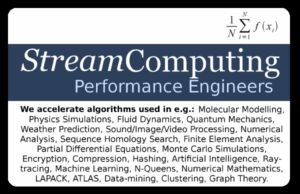Did you find your specialism in the list? The formula is the easiest introduction to GPGPU I could think of, including the need of auto-tuning.

Which algorithms map is best to which accelerator? In other words: What kind of algorithms are faster when using accelerators and OpenCL/CUDA?

Professor Wu Feng and his group from VirginiaTech took a close look at which types of algorithms were a good fit for vector-processors. This resulted in a document: “The 13 (computational) dwarves of OpenCL” (2011). It became an important document here in StreamHPC, as it gave a good starting point for investigating new problem spaces.

The document is inspired by Phil Colella, who identified seven numerical methods that are important for science and engineering. He named “dwarves” these algorithmic methods. With 6 more application areas in which GPUs and other vector-accelerated processors did well, the list was completed.

As a funny side-note, in Brothers Grimm’s “Snow White” there were 7 dwarves and in Tolkien’s “The Hobbit” there were 13.

# The computational dwarves of OpenCL and CUDA

Each part has a description of the “computational dwarf”, examples of application areas and some words from the OpenCL perspective. It is not intended to be complete. It is rather a starting point. You will notice an overlap, which is normal if you take in to account that some algorithms have aspects of two or more. This also implies that some problems have now more solutions.

##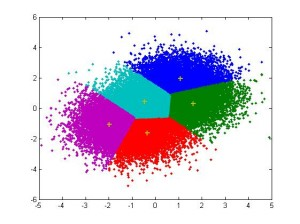Dense Linear Algebra

The classic vector and matrix operations, traditionally divided into Level 1 (vector/vector), Level 2 (matrix/vector), and Level 3 (matrix/matrix) operations. Applications are various:

• Linear algebra: LAPACK, ATLAS.
• Clustering algorithms / Data-mining: StreamCluster, K-means

Normally implemented by loops, and in many cases easy to make parallels in OpenCL.

##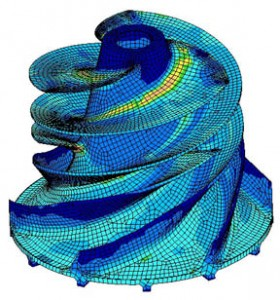Sparse Linear Algebra

Multiplication involving matrices composed primarily of zeroes. Computations can be done much more efficient by moving the non-zero elements are around the diagonal of the matrix.

Applications:

• Finite element analysis
• Partial differential equations

There are two methods using OpenCL. Solve the problem with a series of operations, which results in a large overhead. The second method is using a series of successive approximations, minimising the error-function.

## Spectral MethodsSolving certain differential equations, often involving the use of the Fast Fourier Transform. Spectral methods can be used to solve ordinary differential equations (ODEs), partial differential equations (PDEs) and eigenvalue problems involving differential equations.

Applications:

• Fluid Dynamics
• Quantum Mechanics
• Weather Prediction.

There are various FFT implementation in OpenCL for each hardware architecture. The trick is the tuning.

##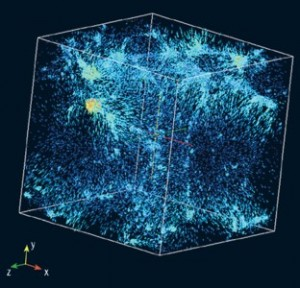N-Body Methods

An N-body simulation is a simulation of a dynamical system of particles, usually under the influence of physical forces, such as gravity. Computations can be done both ways (A influences B the same B influences A) and the state of the whole system is updated after each round. The basic algorithm is O(N^2). Optimizations for larger systems are possible by neighbour-administration and leaving far-away particles out of the computation. Run-time approach-selection is desirable.

Applications:

• Astronomy: cosmology (e.g. formation of galaxies)
• Computational Chemistry: Molecular Dynamics (e.g. protein folding), Molecular modeling
• Physics: Fluid Dynamics, Plasma Physics

OpenCL-implementations can do tens of rounds per second with millions of particles, outperforming scalar implementations with ease.

##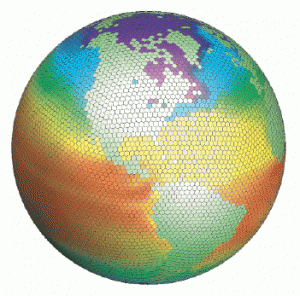Structured Grids

In a structured or regular grid all the elements in the grid have the same dimensions. Think squares and blocks. Computations that depend on neighbors in an irregular grid.

Applications:

• Image Processing: Gaussian image blurring
• Physics Simulations: transient thermal differential equation solver
• Finite Element Method

In OpenCL the grids (of working-groups) are regular too, so mapping is quite easy. The problem remaining to be solved is how to do the communication between the between neighbors.

##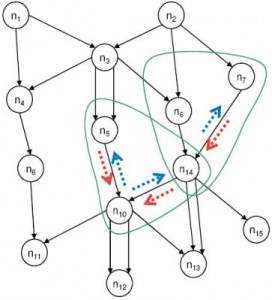Unstructured Grids

All grids that are not regular grids. Different elements have different number of neighbors.

This group has a lot of overlap with backtracking.

Applications:
• Computational fluid dynamics
• Belief propagation

The difficulty here is mapping the irregular grid on the hardware.

## Map-Reduce & Monte Carlo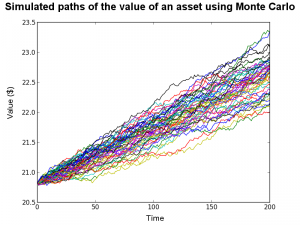Each process runs completely independent from one other, so nearly no communication is required between processes. In case of huge data-sets and compute-intensive algorithms GPUs can be used in combination with Big Data solutions like Hadoop.

Applications:

• Monte-Carlo: computation of pi, portfolio analysis, collision simulation, Sequence Alignment
• Distributed Searching

As communication between the nodes is minimal, this is one of the fastest methods using GPUs.

## Combinational Logic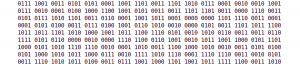These algorithms generally involve performing simple operations on very large amounts of data, which exploit bit-level operations.

Applications:

• Computing checksums, CRCs
• Encryption & Decryption
• Hashing
• Hamming weight

Not all hardware is fit for these types of operations, so device-selection is critical.

##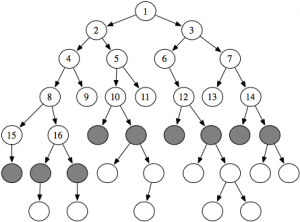Graph Traversal

Graph traversal is the problem of visiting all the nodes in a graph in a particular manner, updating and/or checking their values along the way. Tree traversal is a special case of graph traversal. Has indirect lookups and little computation.

Applications:

• Search: depth-first search, breadth-first search, finding all nodes within one connected component
• Sorting: Quick-sort
• Serialization/Deserialization
• Maze generation
• Collision detection

In OpenCL the trick is to keep the kernels busy enough.

## Dynamic Programming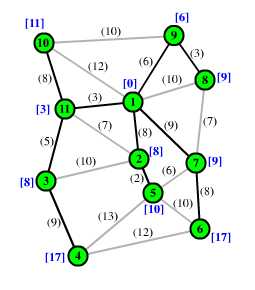This is an algorithmic technique that computes solutions by solving simpler overlapping subproblems. Many dynamic programming problems operate by filling in a grid that represents the solution space, where one location in the grid holds the final answer.

Applications:

• Graph problems: Floyd’s AllPairs, shortest path, Bellman-Ford algorithm
• Sequence alignment: Needleman-Wunsch, Smith-Waterman

As “dynamic” applies, better performance is reached when tuned during run-time.

## Backtracking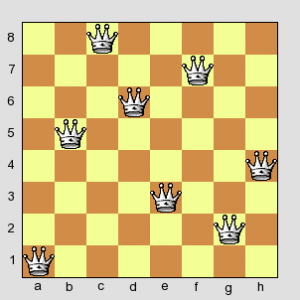This consists in building up all possible solutions and eliminating invalid ones (most times in one step), as there is no overview of all possible solutions when starting. It is effectively a depth-first search of a problem space and therefore a special case of dynamic programming, but with a strong accent on the step-wise creation of the solution-space. The generic solution for this group of algorithms is branch-and-bound. (Divide and conquer!).

Applications:

• Puzzles: N-queens, crosswords, verbal arithmetic, Sudoku, Peg Solitaire.
• Traveling salesman
• Knapsack, subset sum, and partition problems
• Integer Linear Programming
• Boolean Satisfiability
• Combinatorial Optimisation

If the problem-space is expected to be equally divided, a limited number of starting positions is created to walk the problem space in parallel. In OpenCL it is important is to avoid large branches.

## Probabilistic Graphical Models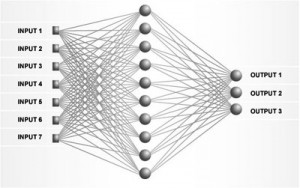A graph that combines uncertainty (probabilities) and logical structure (independence constraints) to compactly represent complex, real-world phenomena.

Applications:

• Bayesian networks: belief networks, probabilistic networks, causal network, knowledge maps
• Hidden Markov models
• Neural networks

As more processes need to update the same node (a typical case for atomics), a lot of time is consumed here.

## Finite State Machines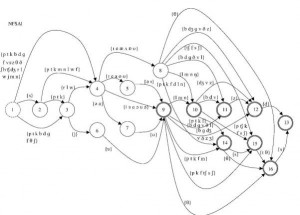A mathematical model of computation used to design both computer programs and sequential logic circuits. It is conceived as an abstract machine that can be in one of a finite number of states.

Applications:

• Video Decoding, Parsing, Compression
• Data Mining
• Find Repeating Patterns

# Why is categorizing important?

Understanding which type of applications perform well makes it easier to decide when to use GPUs and other accelerators, or when to use CPUs or FPGAs.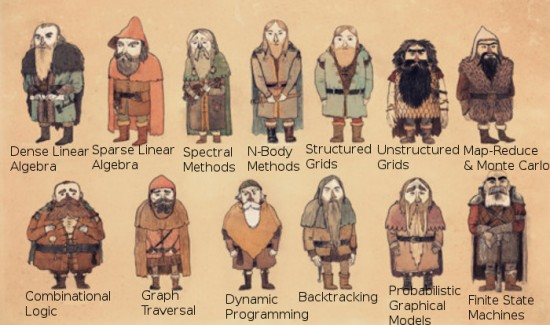As a second step, the right hardware can be better selected when you know the right job category. So don’t just buy a Tesla when you want to start with GPGPU, like others have done. For example, combinational logic is much faster on AMD GPUs. Not all of above algorithms work best on a GPU by definition: OpenCL on a CPU can be a good choice when memory-bandwidth is not the bottleneck (a CPU does ~30GB/s, a GPU up to ~300GB/s).

Along the same line, determining the maximum performance is easier to compare within a group of algorithms. A 4 TFLOPS accelerator can drop to 100 GFLOPS if it is hitting a wall; making it possible to compare apples with apples.

Not being able to map your algorithm to one of the above groups might mean that it would be hard to program in OpenCL or CUDA. Once mapped to a group, optimizations for alike algorithms might also apply to yours… Or it might be, at least, worth investigating.

We hope you have gained some valuable insights and can make steps in your investigation to speed up your software! Remember you can always contact us with comments and questions, or ask our help to make your software optimal.

### Related Posts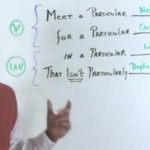#### Commodity and Open Standards – why OpenCL matters

...  it. If you want to know what GPGPU enables, read "The 13 application areas where OpenCL can be used". This also can be applied to CUDA, ...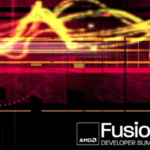#### OpenCL Videos of AMD’s AFDS 2012

...  was full of talks on OpenCL. You missed them, just like me? Then you will be happy that they put ...  robust parallel ...#### Exposing OpenCL on Android: Q&A with Tim Lewis of ZiiLabs

...  usage seems to be media-processing, but what typical applications did you have in mind? (Note: I indirectly ask for the ...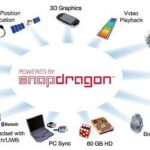#### All the members of the OpenCL working group 2010

...  you're searching for companies who offer OpenCL-products and services, please visit OpenCL:Pro) You probably have ...  to ...